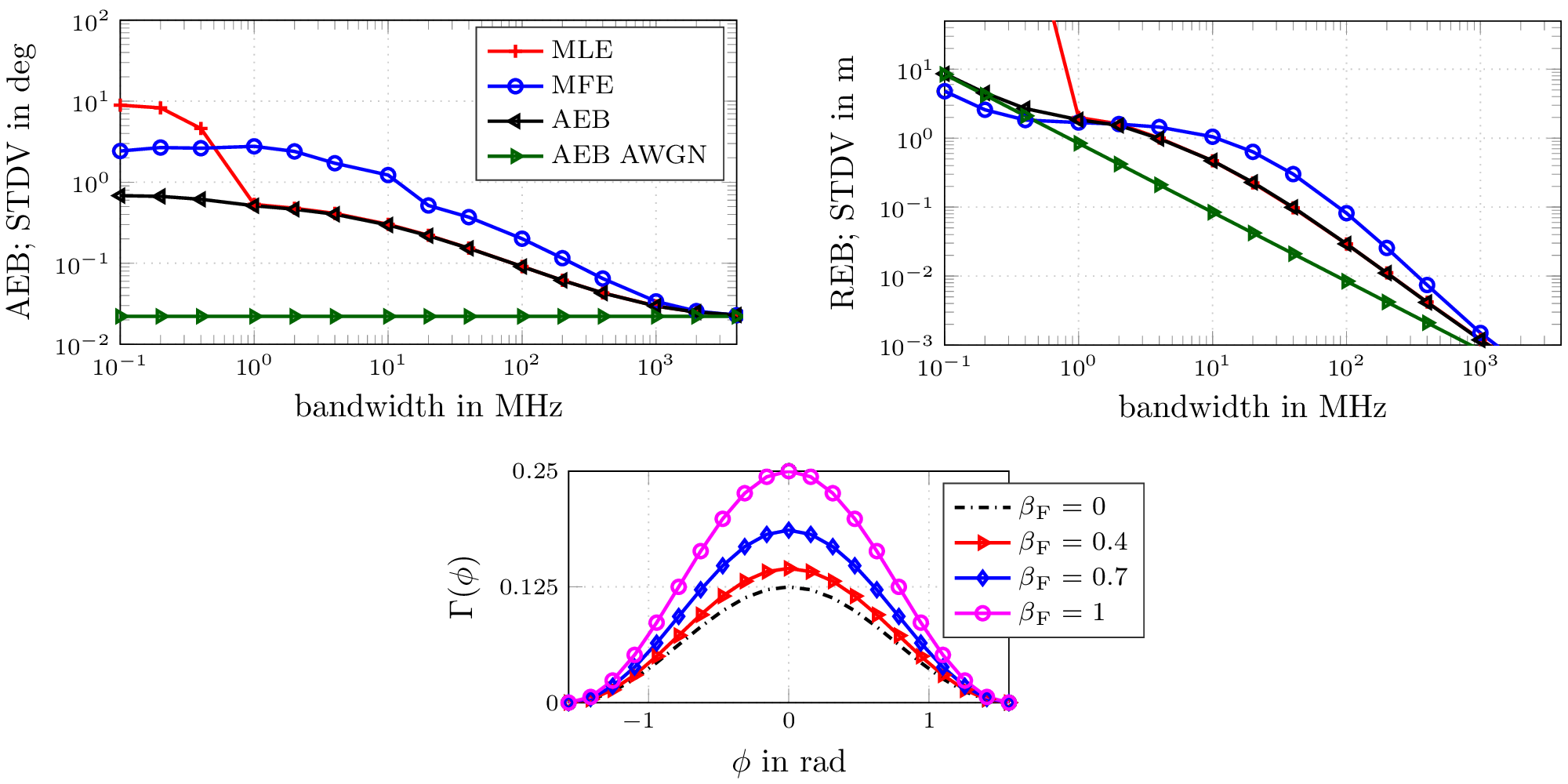Signal Processing and Speech Communication Laboratory

## AoA and ToA Accuracy for Antenna Arrays in Dense Multipath Channels

Published
Fri, Jun 01, 2018
Tags
rotm
ContactThe accuracy that can be achieved in time of arrival (ToA) estimation strongly depends on the utilized signal bandwidth. In an indoor environment multipath propagation usually causes a degradation of the achievable accuracy due to the overlapping signals. A similar effect can be observed for the angle of arrival (AoA) estimation using arrays. This paper derives a closed-form equation for the Cramér-Rao lower bound (CRLB) of the achievable AoA and the ToA error variances, considering the presence of dense multipath. The Fisher information expressions for both parameters allow an evaluation of the influence of channel parameters and system parameters such as the array geometry. Our results demonstrate that the AoA estimation accuracy is strongly related to the signal bandwidth, due to the multipath influence. The theoretical results are evaluated for experimental data, with simulations performed for ULAs with M=2 and M=16 array elements.

The figure illustrates the derived ranging error bound (REB) and the angulation error bound (AEB) in comparison to the resepective AWGN bound. Performance results over different pulse bandwidths are compared for a matched filter estimator (MFE) and a maximum likelihood estimator (MLE) using a ULA with M = 16 array elements and half-wavelength inter-element spacing at a carrier frequency of 7 GHz. It further visualizes the direction dependent factor Γ(Φ) in the derived AEB, again for a ULA with M = 16 and half-wavelength inter-element spacing.